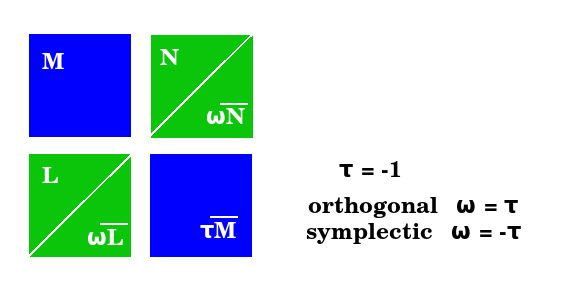# Book: LieAlgebrasChoiceTemplates

Interpret the four classical Lie algebras in terms of the four choice templates.

There are four choice templates:

• {$\prod_{i}(\varnothing + \leftrightarrow_{i})$} Simplexes.
• {$\prod_{i}(\varnothing + (\leftarrow_{i} + \rightarrow_{i}))$} Cross-polytopes.
• {$\prod_{i}((\leftarrow_{i} + \rightarrow_{i})+\leftrightarrow_{i})$} Cubes.
• {$\prod_{i}(\leftarrow_{i} + \rightarrow_{i})$} Cube slices.

The root systems of the classical Lie algebras all include the roots of {$A_{n}$}: {$\pm (x_i-x_j)$}. The other root systems all include the additional roots of {$D_{n}$}: {$\pm (x_i+x_j), i\neq j$}. Finally, {$B_{n}$} includes {$\pm x_i$} and {$C_{n}$} includes {$\pm (x_i+x_i)$}.The Weyl group of a root system is the group generated by the reflections across the hyperplanes of the roots. So the Weyl group is given by the directions of the roots but not their lengths.

 Hyperplane = {$\pm$} Root Transposition {$\pm(e_2-e_1)$} {$e_1 \Leftrightarrow e_2, -e_1 \Leftrightarrow -e_2$} {$\pm(e_2+e_1)$} {$e_1 \Leftrightarrow -e_2, -e_1 \Leftrightarrow e_2$} {$\pm(e_1)$} {$e_1 \Leftrightarrow -e_1$}

There are three ways of defining the reflections {$e_1 \Leftrightarrow -e_1$}:

• {$B_n$}: By swapping with 0. By way of the hyperplane {$\pm(0-e_1)$}. Swapping {$e_1$} with {$0$} as with the cross polytopes. This takes place in the framework {$e_1 - 0$} so the minus sign is a feature of the pairing.
• {$D_n$}: By swapping with any root {$e_2$}. By composing the hyperplanes {$\pm(e_2-e_1)$} and {$\pm(e_2--e_1)$}. Swapping {$e_1$} with {$e_2$} and then {$e_2$} with {$-e_1$}
• {$C_n$}: By swapping directly with {$-e_1$}. By way of the hyperplane {$\pm(e_1--e_1)$}. Swapping {$e_1$} with {$-e_1$} directly. Then there is one minus sign in the pairing, and one carried by {$-e_1$}.

Pairing template

The sign can be attributed to the element or to the pairing template. So try to think that through.

Consider {$X-Y$} as a template where the minus sign imposes an ordering.

• {$A_n$}: {$X, Y$} are taken from {${e_1,e_2 \dots e_n}$} and {$X \neq Y$} is ruled out by {$X-Y=0$}.

Consider {${X,Y}$} as a template where the set indicates inclusion with no ordering.

• {$B_n$}: {$X, Y$} are taken from {${\dots -e_2,-e_1,e_1,e_2 \dots e_n}$} and {$X$} and {$Y$} may refer to the same number so long as they have the same sign, in which case they are understood to coincide.
• {$D_n$}: {$X, Y$} are taken from {${\dots -e_2,-e_1,e_1,e_2 \dots e_n}$} and {$X$} and {$Y$} must refer to different numbers.
• {$C_n$}: {$X, Y$} are taken from {${\dots -e_2,-e_1,e_1,e_2 \dots e_n}$} and {$X$} and {$Y$} may refer to the same number so long as they have the same sign, in which case they are understood to add.

Not satisfactory. :(

Orthogonalities

• Real (orthogonal), complex (unitary), quaternion (symplectic)

• How are the Weyl groups related to the choice templates? Use the relationships between the choice templates and the Weyl groups, and the Weyl groups and the root systems, to relate the root systems and the choice systems. Note the problematic identification as I had expected to identify the coordinate systems with {$C_n$} but their symmetry group instead may match that of {$D_n$}.
• Interpret the three ways of defining the reflection {$e_{i} \leftrightarrow -e_{i}$}. How is this related to turning around the counting?
• What is the geometric relation between {$B_n$} and {$C_n$}?